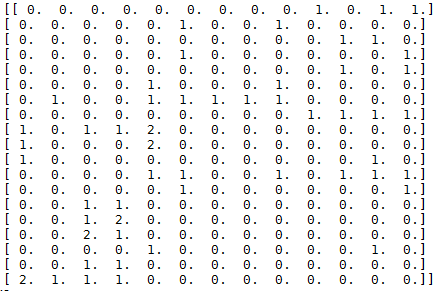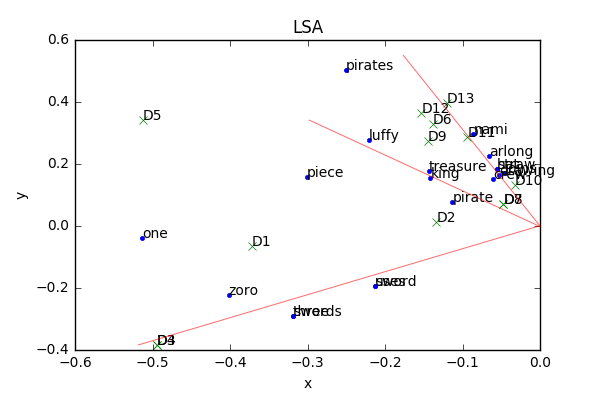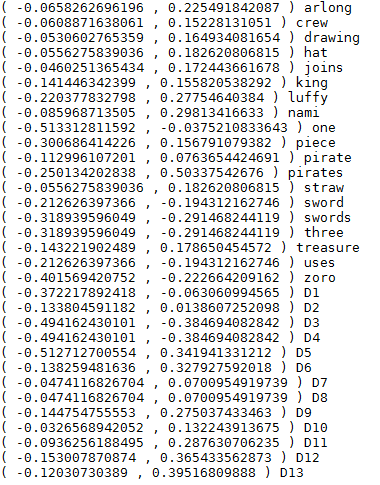# 什么是LSA

LSA(Latent Semantic Analysis), 潜在语义分析。试图利用文档中隐藏的潜在的概念来进行文档分析与检索，能够达到比直接的关键词匹配获得更好的效果。

# LSA可以完全解决上述问题吗？

LSA可以很好的应对上述问题一，对于问题二不能彻底解决。

# LSA的核心思想LSA试图将原始矩阵降维到一个潜在的概念空间（维度不超过 $n$ ），然后每个单词或文档都可以用该空间下的一组权值向量（也可认为是坐标）来表示，这些权值反应了与对应的潜在概念的关联程度的强弱。

$$X = U{\Sigma}V^T$$

$\Sigma$ 矩阵中对角线上的每一个值就是SVD过程中得到的奇异值，其大小反映了其对应的潜在概念的重要程度。

$$X_k = U_k\Sigma_kV_k^T$$

$X_k$ 则是将 $X$ 降维到 $k$ 维的近似结果，这个 $k$ 越接近 $n$, $X_k$ 与 $X$ 也越接近，但我们的目标并不是越接近越好，LSA认为 $k$ 值不宜过大（保留了冗余的潜在概念）也不宜过小。

# LSA结果分析# 参考资料

1 Indexing by Latent Semantic Analysis by Scott Deerwester